# Bihar Board 12th Chemistry Objective Answers Chapter 4 Chemical Kinetics

Bihar Board 12th Chemistry Objective Questions and Answers

## Bihar Board 12th Chemistry Objective Answers Chapter 4 Chemical Kinetics

Question 1.
When a chemical reaction takes place, during the course of the reaction the rate of reaction
(a) keeps on increasing with time
(b) remains constant with time
(c) keeps on decreasing with time
(d) shows irregular trend with time
(c) keeps on decreasing with time

Question 2.
Consider the reaction :
$$2 \mathrm{N}_{2} \mathrm{O}_{4} \rightleftharpoons 4 \mathrm{N} \mathrm{O}_{2}$$ $$\frac{\mathrm{d}\left[\mathrm{N}_{2} \mathrm{O}_{4}\right]}{\mathrm{dt}}=\mathrm{k} \text { and } \frac{\mathrm{d}\left[\mathrm{NO}_{2}\right]}{\mathrm{dt}}=k^{\prime}$$ then
(a) 2k = k’
(b) k = 2k’
(c) k = k’
(d) k = 1/4 k’
(b) k = 2k’Question 3.
For the reaction 2NH3 →N2 + 3H2, if –$$\frac{\mathrm{d}\left[\mathrm{NH}_{3}\right]}{\mathrm{dt}}=\mathrm{k}_{1}\left[\mathrm{NH}_{3}\right], \frac{\mathrm{d}\left[\mathrm{N}_{2}\right]}{\mathrm{dt}}$$ then the relation between k1, k2 and k3 is ………….
(a) k1= k2 = &3
(b) k1 = 3 k2 = 2 k2
(c) 1.5k1 = 3k2 = k3
(d) 2k1= k2 = 3k3
(c) 1.5k1 = 3k2 = k3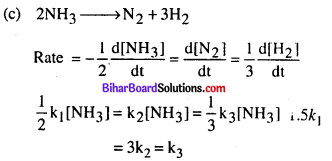Question 4.
The rate of disappearance of SO2 in the reaction, 2SO2 + O2 → 2SO3 is 1.28 x 10-5 M s-1. The rate of appearance of SO3 is …………..
(a) 64 x 10-5 M s-1
(b) 0.32 x 10-5 M s-1
(c) 2.56 x 10-5 Ms-1
(d) 1.28 x 10-5 M s-1
(d) 1.28 x 10-5 M s-1

Question 5.
In a reaction 2X → Y, the concentration of X decreases from 3.0 moles/litre to 2.0 moles/litre in 5 minutes. The rate of reaction is ………………..
(a) 1 mol L-1 min-1
(b) 5 mol L-1 min-1
(c) 1 mol L-1 min-1
(d) 0.5 mol L-1 min-1
(a) 1 mol L-1 min-1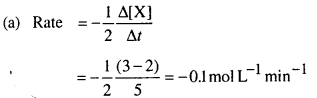Negative sign signifies the decrease in concentration

Question 6.
The rate constant of a reaction depends upon
(a) temperature of the reaction
(b) extent of the reaction
(c) initial concentration of the reaction
(d) the time of completion of reaction
(a) temperature of the reactionQuestion 7.
The chemical reaction, 2O3 → 3O2 proceeds as
$$\mathbf{O}_{3} \rightleftharpoons \mathbf{O}_{2}+[\mathbf{O}] \text { (fast) }$$ [O] + O3 → 2O2 (slow) The rate law expression will be ………….
(a) Rate = k[O] [O3]
(b) Rate = k[O3]2 [O2]-1
(c) Rate = it [O3]2
(d) Rate = k [O2] [O]
(b) Rate = k[O3]2 [O2]-1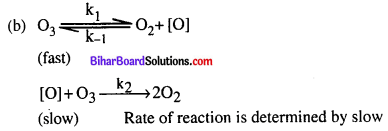[O] is unstable intermediate so substitute the value of
[O] in above equation.
Rate of forward reaction = k1 [O3]
Rate of backward reaction = [k-1] [O2] [O]
At equilibrium,
Rate of forward reaction = Rate of backward reactionQuestion 8.
Rate constant in case of first order reaction is
(a) inversely proportional to the concentration units
(b) independent of concentration units
(c) directly proportional to concentration units
(d) inversely proportional to the square of concentration units
(b) independent of concentration unitsQuestion 9.
The rate constant for the reaction, 2N2Os → 4NO2 + O2 is 2 x 105s-1. If rate of reaction is 1.4 x 10-5 mol L-1 s-1, what will be the concentration of N2O5 in mol L-1?
(a) 8
(b) 0.7
(c) 1.2
(d) 1
(b) 0.7
(b) Rate = k[N2O5] (first order as unit of rate constant is s-1)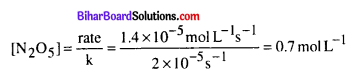Question 10.
The order of reaction is decided by…………..
(a) temperature
(b) mechanism of reaction as well as relative concentration of reactants
(c) molecularity
(d) pressure
(b) mechanism of reaction as well as relative concentration of reactants

Question 11.
Which of the following is an example of a fraction order reaction ?
(a) NH4NO2 → N2 +2H2O
(b) NO + O3 → NO2+O2
(c) 2NO + Br2 → 2NOBr
(d) CH3CHO → CH4 + CO
(d) CH3CHO → CH4 + CO

Question 12.
What will be the rate equation for the reaction 2X + Y→ Z, if the order of the reaction is zero ?
(a) Rate = k[X] [Y]
(b) Rate = k
(c) Rate = A[X]0[Y]
(d) Rate = k[X][Y]0
(b) Rate = kQuestion 13.
For a reaction, I+ OCl → IO + Cl in an aqueous medium, the rate of reaction is given by $$\frac{\mathrm{d}\left[\mathrm{I} \mathrm{O}^{-}\right]}{\mathrm{dt}}=\mathrm{k} \frac{\left[\mathrm{I}^{-}\right]\left[\mathrm{OCl}^{-}\right]}{\left[\mathrm{OH}^{-}\right]}$$ is
(a) – 1
(b) 0
(c) 1
(d) 2
(c) 1

Question 14.
The unit of rate constant for the reaction, 2H2 + 2NO → 2H2O + N2 which has rate – k[H2][NO]2, is …………..
(a) molL1s-1
(b) s-1
(c) mol1L2s-1
(d) mol L-1
(c) mol1L2s-1

(c) Unit of rate constant for the reaction is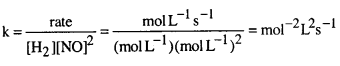Question 15.
The unit of rate and rate constant are same for a…………….
(a) zero order reaction
(b) first order reaction
(c) second order reaction
(d) third order reaction
(a) zero order reaction

Question 16.
The number of molecules of the reactants taking part in a single step of the reaction is indicative of
(a) order of a reaction
(b) molecularity of a reaction
(c) fast step of the mechanism of a reaction
(d) half-life of the reaction
(b) molecularity of a reactionQuestion 17.
The overall rate of a reaction is governed by
(a) the rate of fastest intermediate step
(b) the sum of the rates of all intermediate steps
(c) the average of the rates of all the intermediate steps
(d) the rate of slowest intermediate step.
(d) the rate of slowest intermediate step.

Question 18.
The expression to calculate time required for completion of zero order reaction is ………..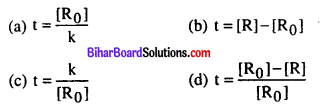(a)

Question 19.
A plot, of log (a -x) against time t is a striaght line. This indicates that the reaction is of
(a) zero order
(b) first order
(c) second order
(d) third order
(b) first order

Question 20.
Radioactive disintegration is an example of ……………
(a) zero order reaction
(b) first order reaction
(c) second order reaction
(d) third order reaction
(b) first order reactionQuestion 21.
The rate constant of a first order reaction is 15 x 10-3 s-1. How long will 5.0 g of this reactant take to reduce to 3.0 g ?
(a) 07 s
(b) 7.57 s
(c) 10.10 s
(d) 15 s
(a) 07 sQuestion 22.
A first order reaction is 20% complete in 10 minutes. What is the specific rate constant for the reaction ?
(a) 0970 min-1
(b) 0.009 min-1
(c) 0.0223 min-1
(d) 2.223 min-1
(c) 0.0223 min-1

Question 23.
A first order reaction takes 40 min for 30% decomposition. What will be t1/2 ?
(a) 7 min
(b) 52.5 min
(c) 46.2 min
(d) 22.7 min
(a) 7 min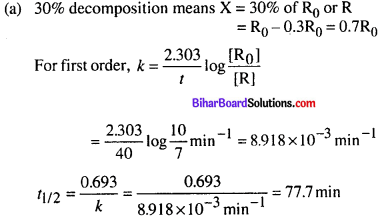Question 24.
A first order reaction has a rate constant 1.15 x 10-3 s-1. How long will 5 g of this reaction take to reduce to 3 g ?
(a) 444 s
(b) 400 s
(c) 528 s
(d) 669 s
(a) 444 s

Question 25.
Half-life period of a first order reaction is 10 min. What percentage of the reaction will be completed in 100 min ?
(a) 25%
(b) 50%
(c) 99.9%
(d) 75%
(c) 99.9%Question 26.
The rate constant for a first order reaction is 2 x 10-2 min-1. The half-life period of reaction is
(a) 69.3 min
(b) 34.65 min
(c) 17.37 min
(d) 3.46 min
(b) 34.65 min

Question 27.
The value of rate of a pseudo first order reaction depends upon
(a) the concentration of both the reactants present in the reaction
(b) the concentration of the reactant present in small amount
(c) the concentration of the reactant present in excess
(d) the value of ΔH of the reaction
(b) the concentration of the reactant present in small amount

Question 28.
Under what conditions a bi-molecular reaction may be kinetically of first order ?
(a) When both reactants have same concentration
(b) When one of the reacting species is in large excess
(c) When the reaction is at equilibrium
(d) When the activation energy of reaction is less
(b) When one of the reacting species is in large excess

Question 29.
The hydrolysis of ethyl acetate, ……………….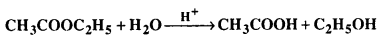(a) zero order
(b) pseudo first order
(c) second order
(d) third order
(b) pseudo first orderQuestion 30.
The temperature dependence of the rate of a chemical reaction can be explained by Arrhenius equation which is…..
(a) k = AeEa/RT
(b) k = Ae-Ea/RT
(c) K = Ae x $$\frac{\mathrm{E}_{\mathrm{a}}}{\mathrm{RT}}$$
(d) K = Ae x $$\frac{R T}{E_{a}}$$
(a) k = AeEa/RT

Question 31.
The energy diagram of a reaction P + Q → R + Sis given. What are A and B in the graph ?(a) A → activation energy, B → heat of reaction
(b) A → threshold energy, B →heat of reaction
(c) A → heat of reaction, B → activation energy
(d) A →potential energy, B → energy of reaction
(a) A → activation energy, B → heat of reaction

Question 32.
For an endothermic reaction, AH represents the enthalpy of the reaction in kj mol-1. The minimum amount of activation energy will be
(a) less than zero
(b) equal to ΔH
(c) less than ΔH
(d) more than ΔH
(d) more than ΔH

Question 33.
The decomposition of a hydrocarbon follows the equation k = (4.5 x 1011 s-1) e-28000 K/T. What will be the value of activation energy ?
(a) 669 kJ mol-1
(b) 232.79   kJ mol-1
(c) 4.5 x 10-1 kJ mol-1
(d) 28000 kJ mol-1
(b) 232.79   kJ mol-1(b) Arrhenius equation, k = Ae Ea/RT
Given equation is k = (4.5x 1011 s-1)e-28000 K/T
Comparing both the equations, we get
$$=\frac{E_{a}}{R T}=-\frac{28000 K}{T}$$
Ea = 28000 K x R = 280000 K x 8.314JK-1mol-1
= 232.79 kJ mol-1

Question 34.
The rate constant for a first order reaction at 300°C for which Ea is 35 kcal mol-1 and frequency constant is 1.45 x 1011 s-1 is
(a) 10 x 10-2s-1
(b) 5.37 x 1010s-1
(c) 5 x 10-4s-1
(d) 7.94 x 10-3s-1
(d) 7.94 x 10-3s-1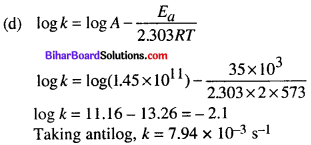Question 35.
The increase in concentration of the reactants lead to change in
(a) ΔH
(b) collision frequency
(c) activation energy
(d) equilibrium constant
(b) collision frequencyQuestion 36.
The rate constant is given by the equation k = P.Ze-Ea/RT  Which factor should register a decrease for the reaction to proceed more rapidly ?
(a) T
(b) Z
(c) E
(d) P
(c) E

Question  37.
Threshold energy is equal to ……………
(a) activation energy
(b) activation energy – energy of molecules
(c) activation energy + energy of molecules
(d) None of these
(c) activation energy + energy of molecules

Question 38.
The role of a catalyst is to change………………..
(a) Gibbs energy of reaction
(b) enthalpy of reaction
(c) activation energy of reaction
(d) equilibrium constant
(c) activation energy of reaction

Question 39
In the presence of a catalyst, the heat evolved or absorbed during the reaction…………..
(a) increases
(b) decreases
(c) remains unchanged
(d) may increase or decrease